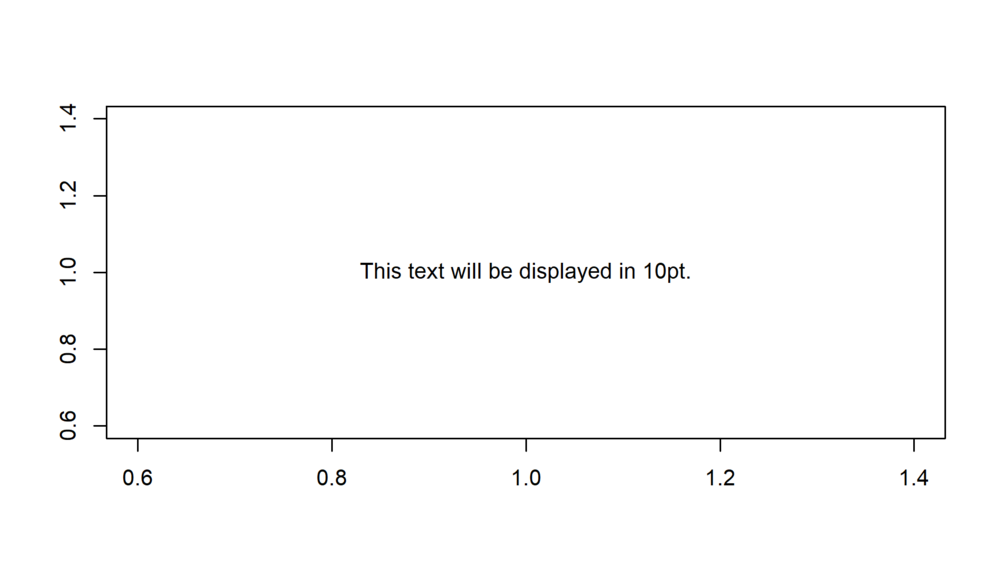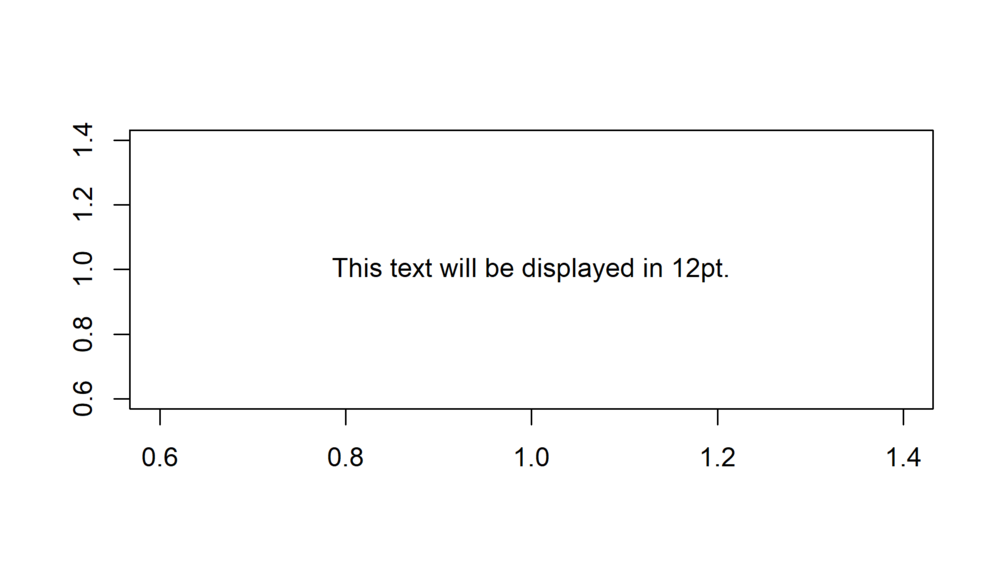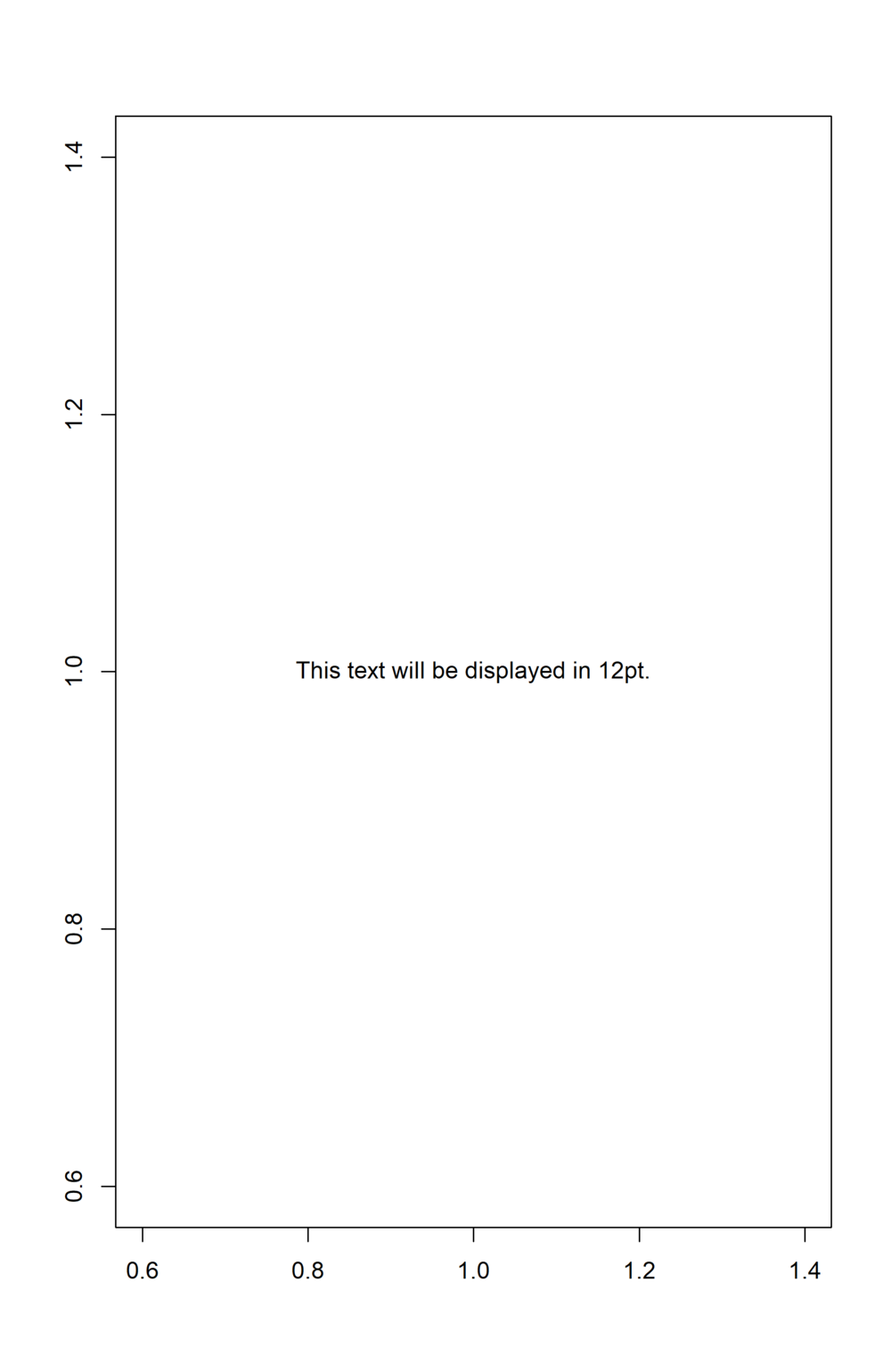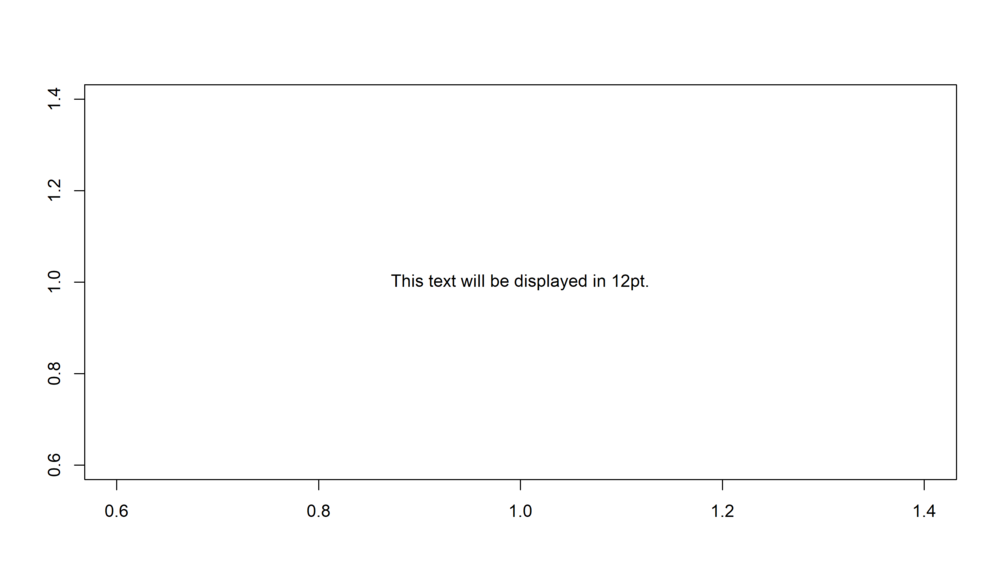## Exporting graphs with fplot

Exporting a graph is often a chore, especially if you want to have the best looking graphs in your publications. One common problem is that it is usually difficult to predict how the text contained in the graph will look once in its final location (i.e. in your document). You may end up with text looking either too big or too small.

fplot provides a set of tools to simplify graph exportation. This is achieved thanks to a different philosophy from existing export functions: instead of giving the target size of the figure (width/height), just give the desired point size of the text. The graph will be created such that once it’s in your final document, the size of the text in the graph will be equal to the desired point size.

In essence, it’s a reparametrization of existing export functions. Below a simple example is detailed.

## Example

You can export any kind of graph with the functions export_graph_start() and export_graph_end().

But first things first, you need to provide the size the image will take in your final document.

By default, the target location of the graph is a US letter page with “normal” margins (i.e. 2.5cm left and right, 2cm top and bottom). You can change it globally with the function setFplot_page. Let’s change it to A4 and one inch margins on all sides:

library(fplot)
setFplot_page(page = "a4", margins = 1)

Now let’s go for the first export:

export_graph_start("first_export.pdf")
plot(1, 1, type = "n", ann = FALSE)
text(1, 1, "This text will be displayed in 10pt.")
export_graph_end()

You can notice that in the end of the export, export_graph_end is used in lieu of dev.off (which must be familier to the ones used to export with base R tools). This function does the same thing as dev.off but also displays the exported graph in the Viewer pane of RStudio or VSCode (provided you use it). This way you can directly see how the exported graph looks.

What about the geometry of the graph? By default the width of the plot is 100% of the text width. You can change it with the width argument: e.g. using relative widths width = 0.5 (50% of the text width), width = "50%" (idem), or absolute widths width = "10cm", width = "5in", etc.

The width to height ratio can be modified with the w2h argument (or you can use h2w for the inverse ratio). The default is w2h = 1.75 (close to the Golden ratio).

Finally the default point size is pt = 10. If embedded in an A4 page with 1 inch margins on both sides, the text of the previously exported graph would be in 10pt:Figure 1: Text in 10pt.

If you increase the pt size that’s what you would get:

export_graph_start("second_export.pdf", pt = 12)
plot(1, 1, type = "n", ann = FALSE)
text(1, 1, "This text will be displayed in 12pt.")
export_graph_end()Figure 2: Text in 12pt.

As we can see, with a larger font any text is larger, and since there is text in the margins, the plotting region becomes smaller.

With this way of exporting, you need to worry only on two things:

1. the final text size of the graph (and you should be worried about it anyway) that you can provide explicitly,
2. the width to height ratio of the graph.

To create the same graph as before but which would take the total height of the page, just use the argument height = 1 (height = 100% or height = 1th, th meaning text height would also work):

export_graph_start("third_export.pdf", pt = 12, height = 1)
plot(1, 1, type = "n", ann = FALSE)
text(1, 1, "This text will be displayed in 12pt.")
export_graph_end()Figure 3: Text in 12pt, total page height.

Finally if you use sideways = TRUE, the settings become width = 1th (100% text height) and height = 0.9tw (90% text width), i.e. the graph would take the full page except a 10% space left for the graph legend.

# You can also set the point size globally
setFplot_page(pt = 12)
export_graph_start("fourth_export.pdf", sideways = TRUE)
plot(1, 1, type = "n", ann = FALSE)
text(1, 1, "This text will be displayed in 12pt.")
export_graph_end()Figure 4: Text in 12pt, sideways.

# Changelog

• version 1.1.0: export_graph_start and export_graph_end replace the family of functions *_fit and fit.off().